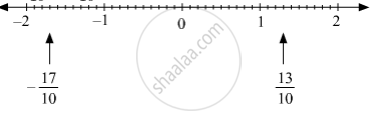# Show the Following Numbers on a Number Line. Draw a Separate Number Line for Each Example. 13 10 , − 17 10 - Mathematics

Sum

Show the following numbers on a number line. Draw a separate number line for each example.

13/10 , (-17)/10

#### Solution

13/10 , -17/10 can be represented on the number line as follows.Concept: Rational Numbers on a Number Line
Is there an error in this question or solution?

#### APPEARS IN

Balbharati Mathematics 8th Standard Maharashtra State Board
Chapter 1 Rational and Irrational numbers
Practice Set 1.1 | Q 1.4 | Page 2